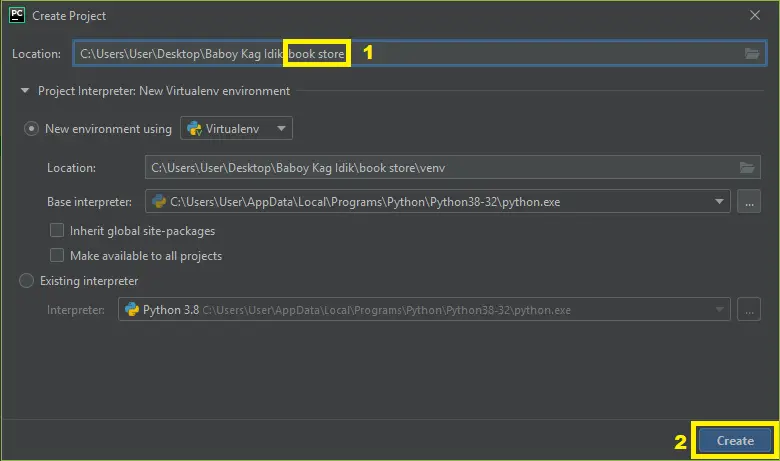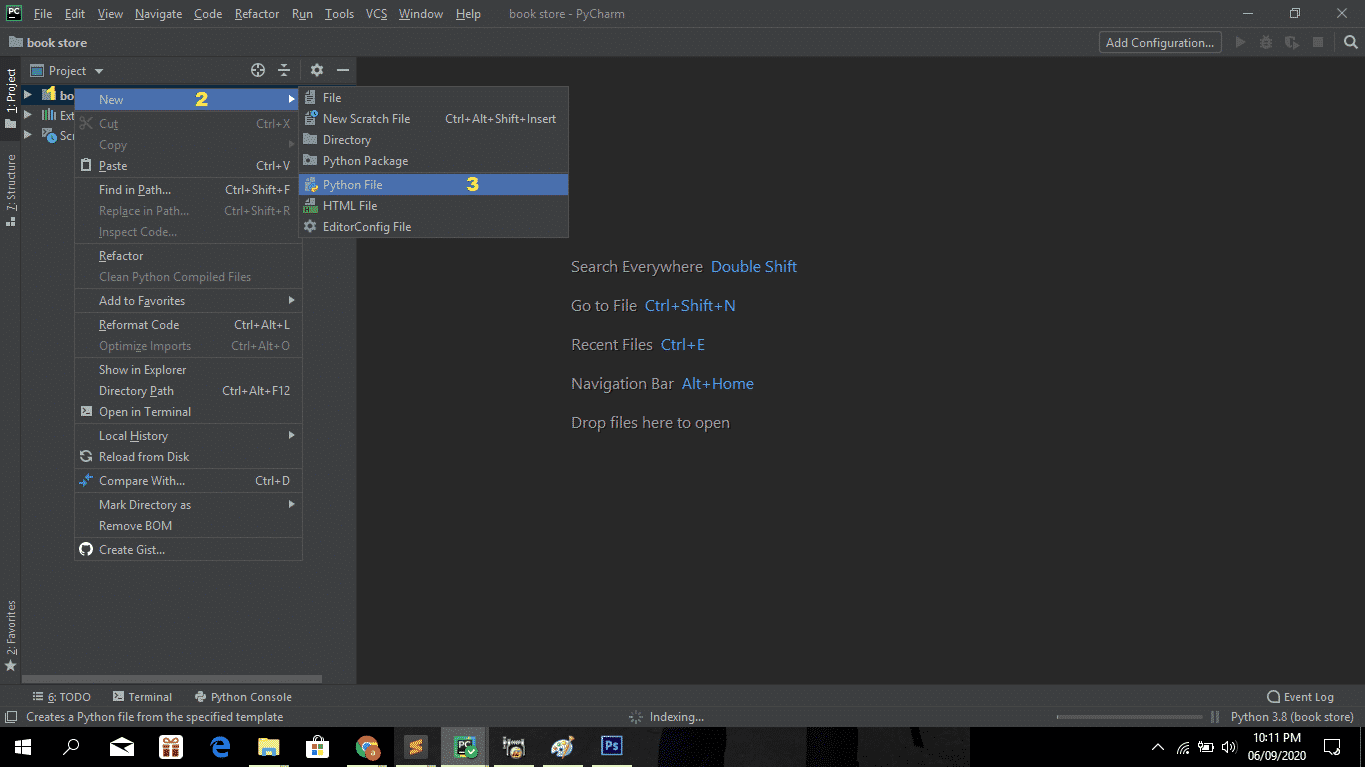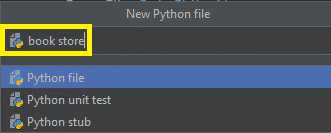# Book Store Management System Python Project With Source Code

The Book Store Management System Python Project is written in Python Programming Language, This Book Store Management System Using Python is an interesting project. The user can add the number of book details and you can see the details stored in the list form.

## Book Store Management System Project in Python – Project Information’s

A Book Shop Management System Project Report about the features of this system, the user can make the list of books with their authors, year, and keep them as records. You just have to type the book information in the text fields and click on the add button to add the information on the record

To start creating a Book Store Management System Python Project, make sure that you have PyCharm IDE installed in your computer.

By the way if you are new to python programming and you don’t know what would be the the Python IDE to use, I have here a list of Best Python IDE for Windows, Linux, Mac OS that will suit for you. I also have here How to Download and Install Latest Version of Python on Windows.

## Steps on how to create a Book Store Management System Python Project

Book Store Management System Python Project With Source Code

• Step 1: Create a project Name.

First open Pycharm IDE and then create a “project name” after creating a project name click the “create” button.• Step 2: Create a python file.

Second after creating a project name, “right click” your project name and then click “new” after that click the “python file“.• Step 3: Name your python file.

Third after creating a python file, Name your python file after that click “enter“.• Step 4: The actual code.

You are free to copy the code given below and download the full source code below.

## The Code Given Below Is For Importing Libraries

``````from tkinter import *
import backend
``````

The code given which is importing the given libraries.

## The Code Given Below Is For The View Command Module

``````def view_command():
list1.delete(0,END)
for row in backend.view():
list1.insert(END, row)``````

IN this module which is the module for view command.

## The Code Given Below Is for Get Selected Row Module

``````def get_selected_row(event):
try:
global select_tup
index=list1.curselection()
select_tup = list1.get(index)
e1.delete(0,END)
e1.insert(END, select_tup)
e2.delete(0,END)
e2.insert(END, select_tup)
e3.delete(0,END)
e3.insert(END, select_tup)
e4.delete(0,END)
e4.insert(END, select_tup)
except IndexError:
pass``````

In this module which is the module for getting the data in database.

## The Code Given Below Is For The Search Module

``````def search_command():
list1.delete(0,END)
for row in backend.search(title_text.get(),author_text.get(),year_text.get(), isbn_text.get()):
list1.insert(END,row)``````

In this module which is the module for searching the data.

## The Code Given Below Is For The Add Book Module

``````def add_book():
backend.insert(title_text.get(),author_text.get(),year_text.get(), isbn_text.get())
list1.delete(0,END)
list1.insert(END,(title_text.get(),author_text.get(),year_text.get(), isbn_text.get()))``````

In this module which is the module for adding the book to database.

## The Code Given Below Is For The Delete Module

``````def delete_book():
backend.delete(select_tup)``````

In this module which is the module for deleting the data from database.

## The Code Given Below Is For The Modify Module

``````def update_book():
backend.update(select_tup, title_text.get(),author_text.get(),year_text.get(), isbn_text.get())``````

In this module which is the module for editing or modifying the data from database.

## Complete Source Code

``````from tkinter import *
import backend
window = Tk()

def get_selected_row(event):
try:
global select_tup
index=list1.curselection()
select_tup = list1.get(index)
e1.delete(0,END)
e1.insert(END, select_tup)
e2.delete(0,END)
e2.insert(END, select_tup)
e3.delete(0,END)
e3.insert(END, select_tup)
e4.delete(0,END)
e4.insert(END, select_tup)
except IndexError:
pass

def view_command():
list1.delete(0,END)
for row in backend.view():
list1.insert(END, row)

def search_command():
list1.delete(0,END)
for row in backend.search(title_text.get(),author_text.get(),year_text.get(), isbn_text.get()):
list1.insert(END,row)

backend.insert(title_text.get(),author_text.get(),year_text.get(), isbn_text.get())
list1.delete(0,END)
list1.insert(END,(title_text.get(),author_text.get(),year_text.get(), isbn_text.get()))

def delete_book():
backend.delete(select_tup)

def update_book():
backend.update(select_tup, title_text.get(),author_text.get(),year_text.get(), isbn_text.get())

window.wm_title("Book Store")

l1 = Label(window, text="Title")
l1.grid(row=0,column=0)

l2 = Label(window, text="Auther")
l2.grid(row=0,column=2)

l3 = Label(window, text="Year")
l3.grid(row=1,column=0)

l4 = Label(window, text="ISBN")
l4.grid(row=1,column=2)

title_text = StringVar()
e1 = Entry(window, textvariable= title_text)
e1.grid(row=0, column=1)

author_text = StringVar()
e2 = Entry(window, textvariable= author_text)
e2.grid(row=0, column=3)

year_text = StringVar()
e3 = Entry(window, textvariable= year_text)
e3.grid(row=1, column=1)

isbn_text = StringVar()
e4 = Entry(window, textvariable= isbn_text)
e4.grid(row=1, column=3)

list1 = Listbox(window, height=6, width=35)
list1.grid(row=2, column =0, rowspan=6, columnspan=2)

list1.bind("<<ListboxSelect>>", get_selected_row)

sb1 =Scrollbar(window)
sb1.grid(row=2, column=2 ,rowspan = 6)

list1.configure(yscrollcommand=sb1.set)
sb1.configure(command=list1.yview)

b1 =Button(window, text= "View All", width=12, command=view_command)
b1.grid(row=2, column=3)

b2 =Button(window, text= "Search Book", width=12, command=search_command)
b2.grid(row=3, column=3)

b3.grid(row=4, column=3)

b4 =Button(window, text= "Update", width=12, command=update_book)
b4.grid(row=5, column=3)

b5 =Button(window, text= "Delete", width=12, command=delete_book)
b5.grid(row=6, column=3)

b6 =Button(window, text= "Close", width=12, command=window.destroy)
b6.grid(row=7, column=3)

window.mainloop()``````

## Summary

The Book Store Management System Python Project is written in Python programming language, Python is very smooth to research the syntax emphasizes readability and it is able to reduces time ingesting in developing.

Also in this tutorial is the simplest way for the beginners or the student to enhance their logical skills in programming. and also in this System project is the way for the students or beginners in designing and developing the system’s.

## Inquiries

1.